# Exams

#### The final exam

The final exam took place on Thursday August 9, at 8:30 AM in the usual lecture Hall E. The Exam scheduleof summer school. The final exam was well solved with 82 average and 12 standard deviation. Below you find the exam with solutions:
The exam, Solutions.
Here were some practice exams: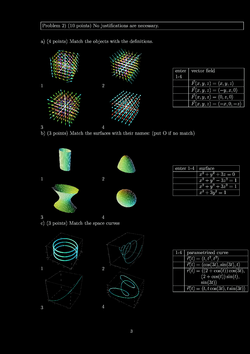Practice I, [Solutions]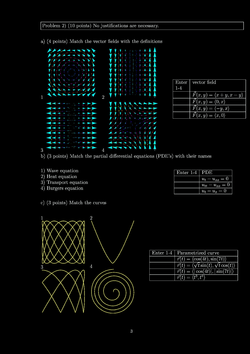Practice II, [Solutions]Practice III, [Solutions]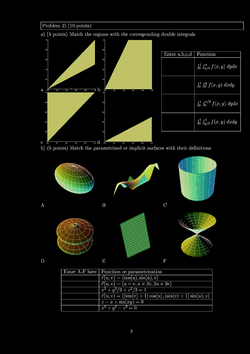Practice IV, [Solutions]Practice V, [Solutions]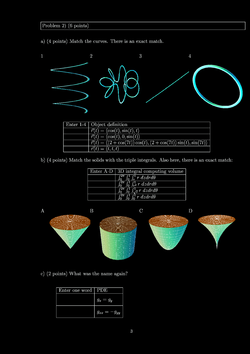Practice VI, [Solutions]

#### Second hourly

The second hourly took place on July 26 2012 in lecture Hall E.

The exam, Solutions.
Here were the practice exams: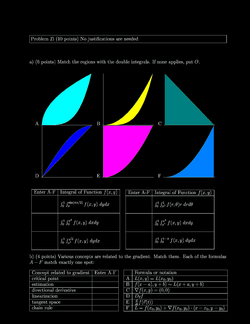Practice I, [Solutions]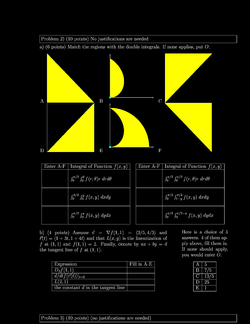Practice II, [Solutions]Practice III, [Solutions]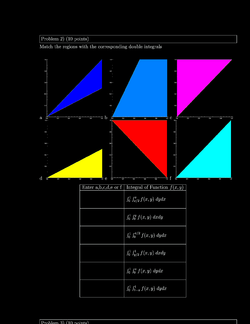Practice IV, [Solutions]

#### First hourlyThe first hourly took place on Thursday July 12, 2012 at the usual place Hall E. The course average was 78 points, the standard deviation 16. We had review on Tuesday. The exam, Solutions.

Here were the practice exams: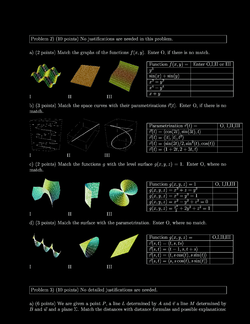Practice I, [Solutions]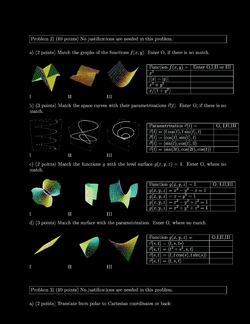Practice II, [Solutions]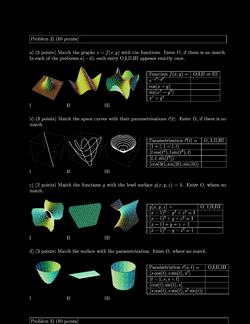Practice III, [Solutions]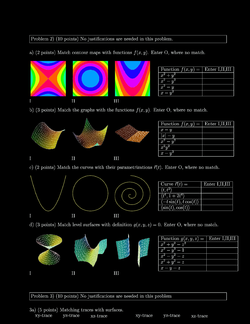Practice IV, [Solutions]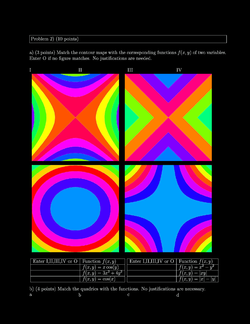Practice V, [Solutions]Practice VI, [Solutions]